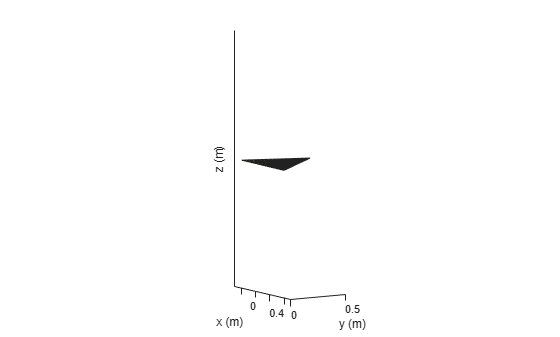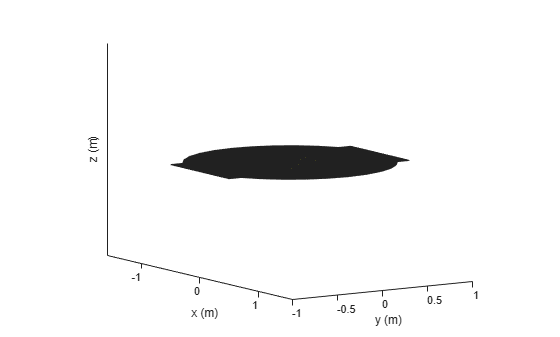# shape.Polygon

Create polygon on xy-plane

Since R2023b

## Description

The `shape.Polygon` lets you create a polygon with metal material properties on the xy-plane, centered at the origin. Use this polygon along with the other shapes and the geometric operations to create a shape for the custom antenna.

## Creation

### Syntax

``poly = shape.Polygon``
``poly = shape.Polygon(Name=Value)``

### Description

````poly = shape.Polygon` creates a polygon shape on the xy-plane.```

example

````poly = shape.Polygon(Name=Value)` sets additional Properties using one or more name-value arguments. `Name` is the property name and `Value` is the corresponding value. You can specify several name-value arguments in any order as `Name1=Value1`, `...`, `NameN=ValueN`. Properties that you do not specify retain their default values.```

## Properties

expand all

Name of the polygon, specified as a string.

Example: `"Polygon1"`

Data Types: `string`

Cartesian coordinates of the polygon vertices, specified as a N-by-3 matrix in meters, where N is the number of points.

Example: `[-1 0 0; -0.5 0.2 0; 0 0 0]`

Data Types: `double`

Metal material of the shape, specified as a metal name string from the Metal Catalog.

Example: `"Copper"`

Data Types: `string`

Color of shape, specified as either a character for color short name or a three-element real vector of RGB triplets. The default shape color is yellow. See the Types of Color Values supported by this property. When you unite two shapes of different colors, use `"Individual"` value to retain the colors of the original shapes in the resultant shape.

Example: `"r"`

Example: `[0 0.447 0.741]`

Data Types: `double` | `string` | `char`

Transparency of shape, specified as a scalar in the (0,1) range, where `0` corresponds to full transparency while `1` corresponds to full opaqueness.

Example: `0.75`

Data Types: `double`

Color of shape edges, specified as either a character for color short name or a three-element real vector of RGB triplets. The default edge color is black. See the Types of Color Values supported by this property. When you unite two shapes of different edge colors, use `"Individual"` value to retain the edge colors of the original shapes in the resultant shape.

Example: `"b"`

Example: `[0.4940 0.1840 0.5560]`

Data Types: `double` | `string` | `char`

## Object Functions

 `add` Boolean unite operation on two shapes `subtract` Boolean subtraction operation on two shapes `intersect` Intersection of shape1 and shape2 `plus` Join two shapes `minus` Carve a shape from other shape `and` Boolean intersection operation on two shapes `area` Calculate area of 2-D shapes in square meters `show` Display antenna, array structures, shapes, or platform `plot` Plot boundary of 2-D shape `mesh` Mesh properties of metal, dielectric antenna, or array structure `rotate` Rotate shape about axis by angle `rotateX` Rotate shape about x-axis by angle `rotateY` Rotate shape about y-axis by angle `rotateZ` Rotate shape about z-axis by angle `translate` Move shape to new location `scale` Change size of shape by fixed factor `imprintShape` Imprint 2-D shape on another shape `createHole` Create a 2-D hole on custom shape `extrude` Extrude 2-D shape on another shape `extrudeLinear` Linearly extrude 2-D shape along specified direction `extrudeRotate` Extrude 2-D shape by angle about z-axis `removeFaces` Interactively select and remove 3-D shape faces `removeSlivers` Remove sliver outliers from boundary of shape

## Examples

collapse all

Create a polygon with vertices at (-0.2,0,0), (0.4,0,0), and (0,0.5,0). Mesh it with a maximum edge length of 6 cm.

```poly = shape.Polygon('Vertices',[-0.2,0,0;0.4,0,0;0,0.5,0]); show(poly)````mesh(poly,MaxEdgeLength=6e-2);`Add a rectangle and circle to create a polygon shape. Mesh it with a maximum edge length of 6 cm.

```polyS = shape.Rectangle + shape.Circle; show(polyS)````mesh(polyS,MaxEdgeLength=6e-2);`## Version History

Introduced in R2023b